## Sample Problems from Chapter 1

Chapter 1: 7 year olds do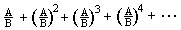It should be noted here that Morris Klein in his book "Mathematics, The Loss of Certainty" describes the confusions of and the mistakes made by, the great mathematicians like Newton, Leibnitz, Euler and the Bernoullis with infinite series and infinite sequences. So I figure if we start young people working on this material, they will make lots of mistakes, but will have a good chance of understanding it later on in high school and college.We're doing important mathematics here, and at the same time we're involving young people in a lot of arithmetic!
1. We're going to add these fractionsforever! Very exciting!
Our strategy will be to add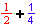, then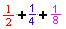, then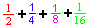and so on, getting these partial sums, until we find a pattern that will help us go forever, without actually doing it.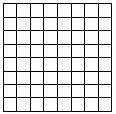Start with the 8x8 square on graph paper, like that at left, and call it one cake (1). Share the cake between 2 people and color in just your share. What part of the cake is this?
Yes,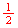(I read this as one-twoth, then one-threeth, and one-fourth, etc. When we say one-half, this doesn't tells how many people are sharing the cake. This is important for young people.)

There are lots of ways to color this in, we'll do it this way:Notice, we'll color in the part of the cake, then write the fraction in the same color.

Now color inofwith a different color, and add it on to the first piece (don't overlap the first one).
What size piece is this new one? How many of these make the whole cake? So the blue piece is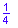of the cake.
Your picture will look something like this: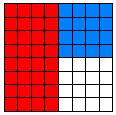Now add.

If you say, I would draw a picture of a cake, cut it into 6 equal pieces, then ask if theis bigger or smaller than.is smaller so it can't be what we are looking for. If you look at the picture carefully, we get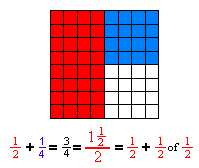Are the pieces we're adding getting bigger or smaller? Are the partial sums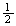, and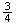getting bigger or smaller or staying the same?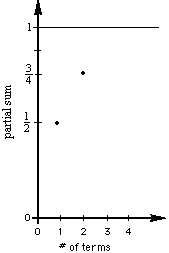Now we'll graph the partial sums vs the number of terms. The first two are shown at the right.

Now takeofoforofthen color it in, get the name of this piece, add the first three fractions, then graph the partial sum. Continue this process to get the next three.
Find patterns in the fractions added.
Find patterns in the partial sums.
Find patterns in the graph of the partial sums.

If we keep taking one-twoth of each piece and adding it, forever, what's going to happen? Will the partial sum ever get to 2? 1? What's the smallest number that will be too big? (Will the 8 by 8 square ever get filled in?) Can you generalize the partial sum?

2. Now try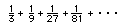use a 9x9 square grid, color in 1/3, then 1/3 of 1/3, etc. Add the fractions as you go. List the partial sums and graph these. Look for patterns. What does this infinite series go to?

3. Try more of these infinite series, then try to generalize to= ? Don's videotape #1  shows Jonathan, at age 7, doing this.

4. Write a program on a calculator or computer to get the infinite series.